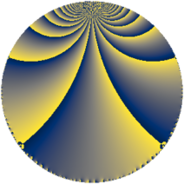# Properties

 Label 252.4.beLevel $252$ Weight $4$ Character orbit 252.be Rep. character $\chi_{252}(107,\cdot)$ Character field $\Q(\zeta_{6})$ Dimension $96$ Newform subspaces $1$ Sturm bound $192$ Trace bound $0$

# Learn more

## Defining parameters

 Level: $$N$$ $$=$$ $$252 = 2^{2} \cdot 3^{2} \cdot 7$$ Weight: $$k$$ $$=$$ $$4$$ Character orbit: $$[\chi]$$ $$=$$ 252.be (of order $$6$$ and degree $$2$$) Character conductor: $$\operatorname{cond}(\chi)$$ $$=$$ $$84$$ Character field: $$\Q(\zeta_{6})$$ Newform subspaces: $$1$$ Sturm bound: $$192$$ Trace bound: $$0$$

## Dimensions

The following table gives the dimensions of various subspaces of $$M_{4}(252, [\chi])$$.

Total New Old
Modular forms 304 96 208
Cusp forms 272 96 176
Eisenstein series 32 0 32

## Trace form

 $$96q + O(q^{10})$$ $$96q - 144q^{13} - 60q^{16} + 480q^{22} + 1032q^{25} + 900q^{28} - 1008q^{34} + 504q^{37} + 492q^{40} + 120q^{46} + 1488q^{49} - 708q^{52} + 1932q^{58} - 2160q^{61} - 2376q^{64} - 588q^{70} + 1320q^{73} - 1368q^{76} - 996q^{82} + 2592q^{85} - 1524q^{88} - 3852q^{94} - 2064q^{97} + O(q^{100})$$

## Decomposition of $$S_{4}^{\mathrm{new}}(252, [\chi])$$ into newform subspaces

Label Dim. $$A$$ Field CM Traces $q$-expansion
$$a_2$$ $$a_3$$ $$a_5$$ $$a_7$$
252.4.be.a $$96$$ $$14.868$$ None $$0$$ $$0$$ $$0$$ $$0$$

## Decomposition of $$S_{4}^{\mathrm{old}}(252, [\chi])$$ into lower level spaces

$$S_{4}^{\mathrm{old}}(252, [\chi]) \cong$$ $$S_{4}^{\mathrm{new}}(84, [\chi])$$$$^{\oplus 2}$$